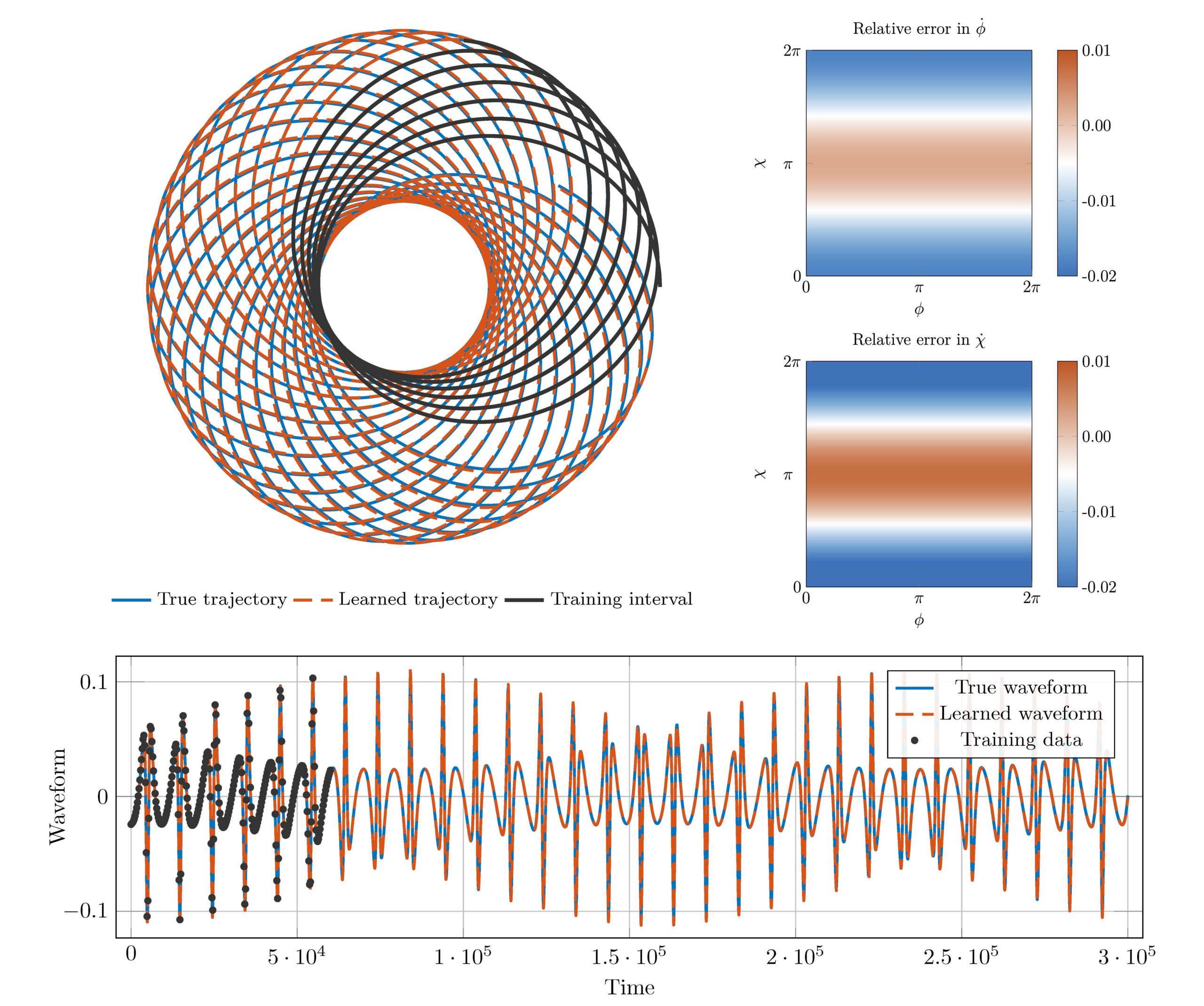### Highlights#### Orbital dynamics of binary black hole systems can be learned from gravitational wave measurements

ICERM - March 2021The collision of massive compact objects, such as black holes (BHs) or neutron stars, emit an immense amount of energy in the form of gravitational waves. With the advent of advanced gravitational-wave detectors, such as the NSF-funded Laser Interferometer Gravitational-Wave Observatory (LIGO), we are now able to directly observe gravitational waves. Encoded in these waves are information about black holes, populations of black holes, and the equations describing gravitational phenomena. Traditional approaches to model gravitational waves and BH dynamics are often obtained by solving simplified forms of the Einstein field equation (a nonlinear, coupled, hyperbolic-elliptic partial differential equations). For example, the Einstein equation may be simplified into a coupled system of ordinary differential equations that in turn describe the BH dynamical motion, which is then used to calculate the emitted gravitational waves.